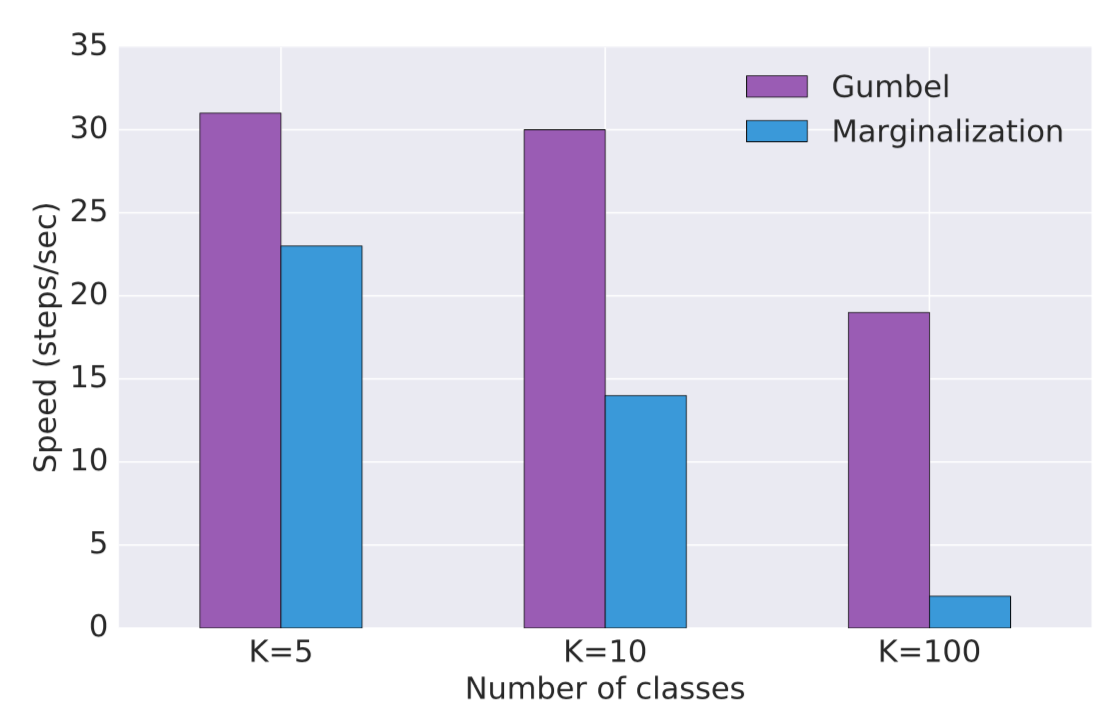Categorical Reparameterization with Gumbel-Softmax@ICLR17.

## Gumbel-Max Trick

The Gumbel-Max trick provides a simple a simple way to draw samples $$\mathbf{z}$$ (one-hot vector) from a categorical distribution with class probabilities $$\mathbf{\pi}$$:

$\mathbf{z} = \text{one-hot}\left(\operatorname{argmax}_i[g_i + \log \pi_i]\right)$

where $$\mathbf{g} = -\log(-\log(\mathbf{u})), \mathbf{u}\sim \text{Uniform}(0, 1)$$.

As the operator $$\operatorname{argmax}$$ is not differentiable we use the $$\operatorname{softmax}$$ function as a continuous, differentiable approximation and generate the (approximated) samples $$\mathbf{z}$$:

$\mathbf{z} = \operatorname{softmax}\left((\log(\pi) + \mathbf{g})/\tau\right)$

where $$\tau$$ is a constant referred as temperature. As the temperature $$\tau$$ approaches 0, samples from the Gumbel-Softmax distribution become one-hot and the Gumbel-Softmax distribution becomes identical to the Categorical distribution.

For learning, there is a tradeoff between small temperatures, where samples are close to one-hot but the variance of the gradients is large, and large temperatures, where samples are smooth but the variance of the gradients is small. In practice, it is usually to start at a high temperature and anneal to a small one.

Under the Gumbel-Softmax distribution, the probability distribution parameter $$\pi$$ becomes the parameter of a deterministic function which allows us to compute its gradient estimation with low variance.

## Review of the reparameterization trick

The reparameterization trick is used when we wish to draw samples $$\mathbf{z}$$ from the distributions $$p_\theta(\mathbf{z})$$ that are reparameterizable and it represents $$\mathbf{z}$$ as a deterministic function of the paramter $$\theta$$ and a random variable $$\epsilon$$ sampling from a fixed distribution, i.e., $$\mathbf{z} = g(\theta, \epsilon)$$ . It transforms the proability distribution parameters into the parameters of a deterministic function $$g$$, if $$g$$ is differentiable we have:

$\frac { \partial } { \partial \theta } \mathbb { E } _ { \mathbf{z} \sim p _ { \theta } } [ f ( \mathbf{z} ) ) ] = \frac { \partial } { \partial \theta } \mathbb { E } _ { \epsilon \sim p_\epsilon} [ f ( g ( \theta , \epsilon ) ) ] = \mathbb { E } _ { \epsilon \sim p _ { \epsilon } } \left[ \frac { \partial f } { \partial g } \frac { \partial g } { \partial \theta } \right]$

## Application in semi-supervised learning

In the paper semi-vae we have the ELBO for the unlabelled data:

$\mathcal { U } ( \mathbf { x } ) = \mathcal { H } \left[ q _ { \phi } ( y \vert \mathbf { x } ) \right] - \sum _ { y } q _ { \phi } ( y \vert \mathbf { x } ) \mathcal { L } ( \mathbf { x } ,y )$

which requires us to marginalize over all $$k$$ classes and will become prohibitively expensive for models with a large number of classes. We can use Gumbel-Softmax distribution to replace the Categorical distribution to get

$\mathcal { U } ( \mathbf { x } ) = \mathcal { H } \left[ q _ { \phi } ( y \vert \mathbf { x } ) \right] - q _ { \phi } ( y \vert \mathbf { x } ) \mathcal { L } ( \mathbf { x } ,y )$Figure MINE Algorithm